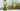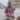# 5 Handy Applications of JavaScript Array.from()

Posted August 27, 2019Start discussion

Any programming language has functions that go beyond the basic usage. It happens thanks to a successful design and a wide area of problems it tries to solve.

One such function in JavaScript is the `Array.from()`: a workhorse allowing lots of useful transformations on JavaScript collections (arrays, array-like objects, iterables like string, maps, sets, etc).

In this post, I will describe 5 use cases of `Array.from()` that are both useful and interesting.

## 1. Quick introduction

Before starting, let’s recall what `Array.from()` does. Here’s how you would call the function:

`javascript`Array.from(arrayLikeOrIterable[, mapFunction[, thisArg]]);``

It’s first obligatory argument `arrayLikeOrIterable` is an array-like object or an iterable.

The second optional argument `mapFunction(item, index) {...}` is a function invoked on every item in the collection. The returned value is inserted into the new collection.

Finally, the third optional argument `thisArg` is used as `this` value when invoking `mapFunction`. This argument is rarely used.

For examples, let’s multiply by 2 the numbers of an array-like object:

`javascript`const someNumbers = { '0': 10, '1': 15, length: 2 };Array.from(someNumbers, value => value * 2); // => [20, 30]``

## 2. Transform array-like into an array

The first useful application of `Array.from()` is indicated directly from its definition: transform an array-like object into an array.

Usually, you meet these strange creatures array-like objects as `arguments` special keyword inside of a function, or when working with DOM collections.

In the following example, let’s sum the arguments of a function:

`javascript`function sumArguments() {  return Array.from(arguments).reduce((sum, num) => sum + num);}sumArguments(1, 2, 3); // => 6``

`Array.from(arguments)` transforms the array-like `arguments` into an array. The new array is reduced to the sum of its elements.

Moreover, you can use `Array.from()` with any object or primitive that implements the iterable protocol. Let’s see a few examples:

`javascript`Array.from('Hey');                   // => ['H', 'e', 'y']Array.from(new Set(['one', 'two'])); // => ['one', 'two']const map = new Map();map.set('one', 1)map.set('two', 2);Array.from(map); // => [['one', 1], ['two', 2]]``

## 3. Clone an array

There is a tremendous number of ways to clone an array in JavaScript.

As you might expect, `Array.from()` easily shallow copies an array:

`javascript`const numbers = [3, 6, 9];const numbersCopy = Array.from(numbers);numbers === numbersCopy; // => false``

`Array.from(numbers)` creates a shallow copy of `numbers` array. The equality check `numbers === numbersCopy` is `false`, meaning that while having the same items, these are different array objects.

Is it possible to use `Array.from()` to create a clone of the array, including all the nested ones? Challenge accepted!

`javascript`function recursiveClone(val) {  return Array.isArray(val) ? Array.from(val, recursiveClone) : val;}const numbers = [[0, 1, 2], ['one', 'two', 'three']];const numbersClone = recursiveClone(numbers);numbersClone; // => [[0, 1, 2], ['one', 'two', 'three']]numbers === numbersClone // => false``

`recursiveClone()` creates a deep clone of the supplied array. This is achieved by calling recursively `recursiveClone()` on array items that are arrays too.

Can you write a shorter than mine version of recursive clone that uses `Array.from()`? If so, please write a comment below!

## 4. Fill an array with values

In case if you need to initialize an array with the same values, `Array.from()` is at your service too.

Let’s define a function that creates an array filled with the same default values:

`javascript`const length = 3;const init   = 0;const result = Array.from({ length }, () => init);result; // => [0, 0, 0]``

`result` contains a new array having `3` items initialized with zeros. This is done by invoking `Array.from()` with an array-like object `{ length }`, and a map function that returns the initialization value.

However, there is an alternative method array.fill() that can be used to achieve the same result:

`javascript`const length = 3;const init   = 0;const result = Array(length).fill(init);fillArray2(0, 3); // => [0, 0, 0]``

`fill()` method fills the array correctly with initialization values, regardless of empty slots.

### 4.1 Fill an array with new objects

When every item of the initialized array should be a new object, `Array.from()` is a better solution:

`javascript`const length = 3;const resultA = Array.from({ length }, () => ({}));const resultB = Array(length).fill({});resultA; // => [{}, {}, {}]resultB; // => [{}, {}, {}]resultA === resultA; // => falseresultB === resultB; // => true``

`resultA` created by `Array.from()` is initialized with different instances of empty objects `{}`. It happens because the map function `() => ({})` on every invocation returns a new object.

However, `resultB` created by `fill()` method is initialized with the same instance of an empty object.

Is it possible to use array.map() method to achieve the same? Let’s try that:

`javascript`const length = 3;const init   = 0;const result = Array(length).map(() => init);result; // => [undefined, undefined, undefined]``

The `map()` approach seems to be incorrect. Instead of the expected array with three zeros, an array with 3 empty slots is created.

It happens because `Array(length)` creates an array having 3 empty slots (also called sparse array), but `map()` method skips the iteration over these empty slots.

## 5. Generate ranges of numbers

You can use `Array.from()` to generate ranges of values. For example, the following function range generates an array with items starting `0` until `end - 1`:

`javascript`function range(end) {  return Array.from({ length: end }, (_, index) => index);}range(4); // => [0, 1, 2, 3]``

Inside `range()` function, `Array.from()` is supplied with the array-like `{ length: end }`, and a map function that simply returns the current index. This way you can generate ranges of values.

## 6. Unique items of an array

A nice trick resulting from the ability of `Array.from()` to accept iterable objects is to quickly remove duplicates from an array. It is achieved in combination with `Set` data structure:

`javascript`function unique(array) {  return Array.from(new Set(array));}unique([1, 1, 2, 3, 3]); // => [1, 2, 3]``

At first, `new Set(array)` creates a set containing the items of the array. Internally, the set removes the duplicates.

Because the set is iterable, `Array.from()` extracts the unique items into a new array.

## 7. Conclusion

`Array.from()` static method accepts array-like objects, as well as iterables. It accepts a mapping function. Moreover, the function does not skip iteration over empty holes. This combination of features gives `Array.from()` a lot of possibilities.

As presented above, you can easily transform array-like objects to arrays, clone arrays, fill arrays with initial values, generates ranges and remove duplicated array items.

Indeed, `Array.from()` is a combination of good design, configuration flexibility allowing a wide area of collection transformations.

What other interesting use cases of Array.from() do you know? Please write a comment below!

## Quality posts into your inbox

I regularly publish posts containing:

• Important JavaScript concepts explained in simple words
• Overview of new JavaScript features
• How to use TypeScript and typing
• Software design and good coding practices